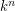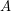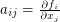# Jacobian Conjecture

 Importance: High ✭✭✭
 Author(s): Keller, Otto-Heinrich
 Subject: Geometry » Algebraic Geometry
 Keywords: Affine Geometry Automorphisms Polynomials
Conjecture   Letbe a field of characteristic zero. A collectionof polynomials in variablesdefines an automorphism ofif and only if the Jacobian matrix is a nonzero constant.
The Jacobian determinant is the determinant of the matrixwith. It is elementary to show that if the mapis an automorphism, then the Jacobian determinant is a nonzero constant, by using the inverse map. The other direction has turned out to be rather difficult.